# Centrality dependence of particle production in p-Pb collisions at $\sqrt{s_{\rm NN} }$= 5.02 TeV

We report measurements of the primary charged particle pseudorapidity density and transverse momentum distributions in p-Pb collisions at $\sqrt{s_{\rm NN}}$ = 5.02 TeV, and investigate their correlation with experimental observables sensitive to the centrality of the collision. Centrality classes are defined using different event activity estimators, i.e. charged particle multiplicities measured in three disjunct pseudorapidity regions as well as the energy measured at beam rapidity (zero-degree). The procedures to determine the centrality, quantified by the number of participants ($N_{\rm part}$), or the number of nucleon-nucleon binary collisions ($N_{\rm coll}$), are described. We show that, in contrast to Pb-Pb collisions, in p-Pb collisions large multiplicity fluctuations together with the small range of participants available, generate a dynamical bias in centrality classes based on particle multiplicity. We propose to use the zero-degree energy, which we expect not to introduce a dynamical bias, as an alternative event-centrality estimator. Based on zero-degree energy centrality classes, the $N_{\rm part}$ dependence of particle production is studied. Under the assumption that the multiplicity measured in the Pb-going rapidity region scales with the number of Pb-participants, an approximate independence of the multiplicity per participating nucleon measured at mid-rapitity of the number of participating nucleons is observed. Furthermore, at high-$p_{\rm T}$ the p-Pb spectra are found to be consistent with the pp spectra scaled by $N_{\rm coll}$ for all centrality classes. Our results represent valuable input for the study of the event activity dependence of hard probes in p-Pb collision and, hence, help to establish baselines for the interpretation of the Pb-Pb data.

Figures

## Figure 1

 Distribution of the sum of amplitudes in the V0A hodoscopes (Pb-going), as well as the NBD-Glauber fit (explained in the text). Centrality classes are indicated by vertical lines. The inset shows a zoom-in on the most peripheral events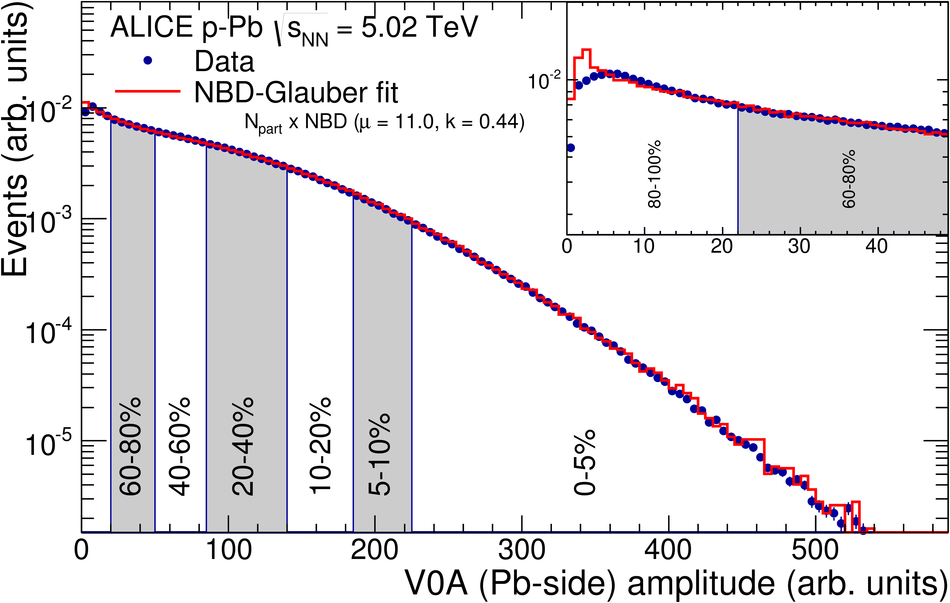## Figure 2

 Values of $\langle \Ncoll \rangle$ extracted from CL1, V0M, V0A, ZNA and by ordering the events according to the impact parameter distribution ($b$). The systematic uncertainty, given by the quadrature sum of the uncertainty from the Glauber parameters and the MC-closure test, are drawn around the values obtained with $b$## Figure 3

 Left: Glauber and Glauber-Gribov Monte Carlo $N_{part}$ distributions for 5.02 TeV p-Pb collisions. Right: Measured V0A distribution compared to Glauber and Glauber-Gribov fits assuming $N_{part}$ or $N_{coll}$ scaling. The inset shows a zoom-in on the most peripheral events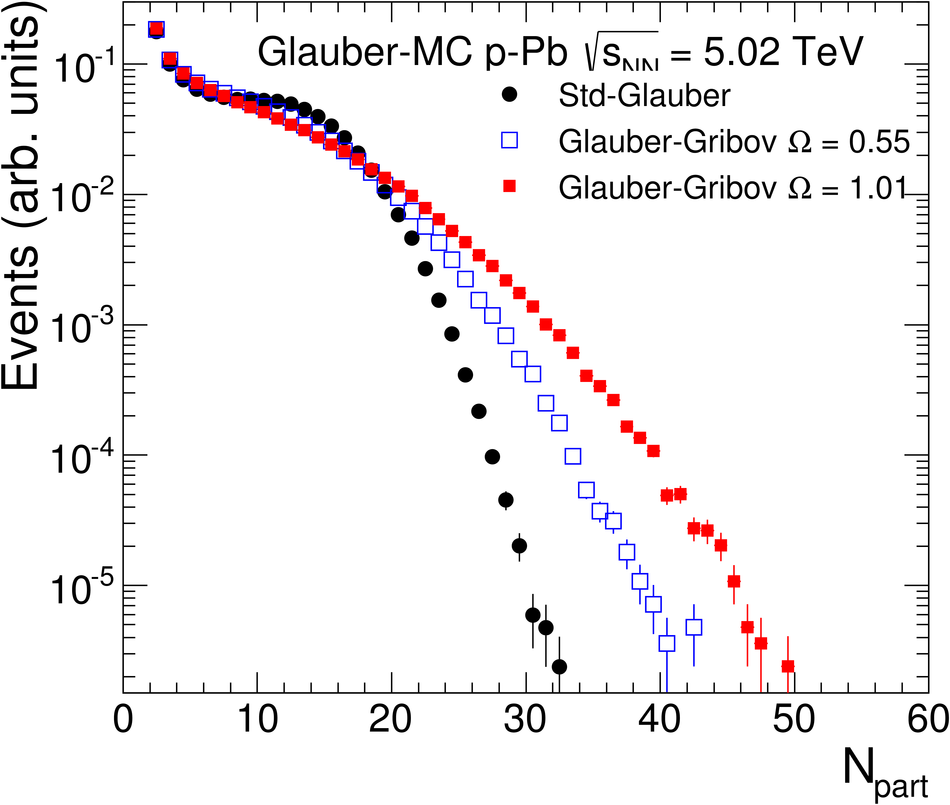## Figure 4

 Left: Correlation between Pb remnant neutron (ZNA) and proton (ZPA) calorimeter energies Right: Average signal on the p remnant side (ZNC) versus averageenergy detected by ZNA in centrality bins selected using ZNA.## Figure 5

 Distribution of the neutron energy spectrum measured in the Pb-remnant side ZN calorimeter The distribution is compared with the corresponding distribution from the SNM-Glauber model (explained in the text) shown as a line. Centrality classes are indicated in the figure. The inset shows a zoom-in on the most peripheral events## Figure 6

 V0A ring1 signal distributions The top left panel shows the distribution for MB events together witha NBD-Glauber fit. The remaining panels show the distributions andmean values for centrality classes selected with ZNA. These arecompared to those obtained by the convolution of the ${\cal P}(\Ncoll| cent_{\rm ZNA})$ distributions from the SNM with the NBD from the NBD-Glauber fit to V0A ring1. Data are also compared to thedistributions obtained with an unfolding procedure, where the $N_{coll}$ distributions have been fitted to the data using the parameters fromthe NBD-Glauber fit. The bottom right panel compares the mean valuesof these distributions as a function of the centrality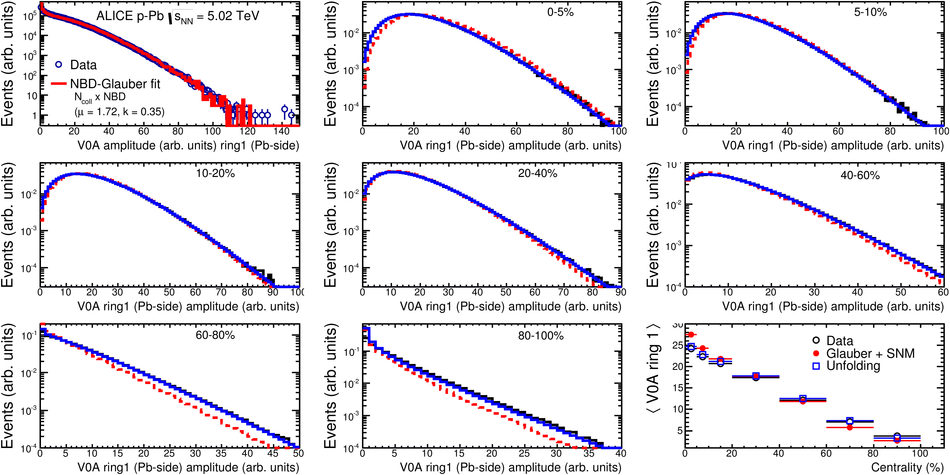## Figure 7

 Top: Scatter plot of number of participating nucleons versus impact parameter; Bottom: Scatter plot of multiplicity versus the number of participating nucleons from the Glauber fit for V0A. The quantities are calculated with a Glauber Monte Carlo of p-Pb (left) and Pb-Pb (right) collisions## Figure 8

 Left: Multiplicity fluctuation bias quantified as the mean multiplicity per $\avNpart / \mu$ from the NBD-Glauber MC in p-Pb and Pb-Pb calculations. Right: Relative width of the NBD obtained from the NBD-Glauber fit of various multiplicity distributions in p-Pb and Pb-Pb calculations## Figure 9

 Number of hard scatterings (MSTI(31) in PYTHIA6) per $\Ncoll$ as a function of the centrality calculated with a toy MC that couples a pp PYTHIA6 calculation to a p-Pb Glauber MC (described in the text).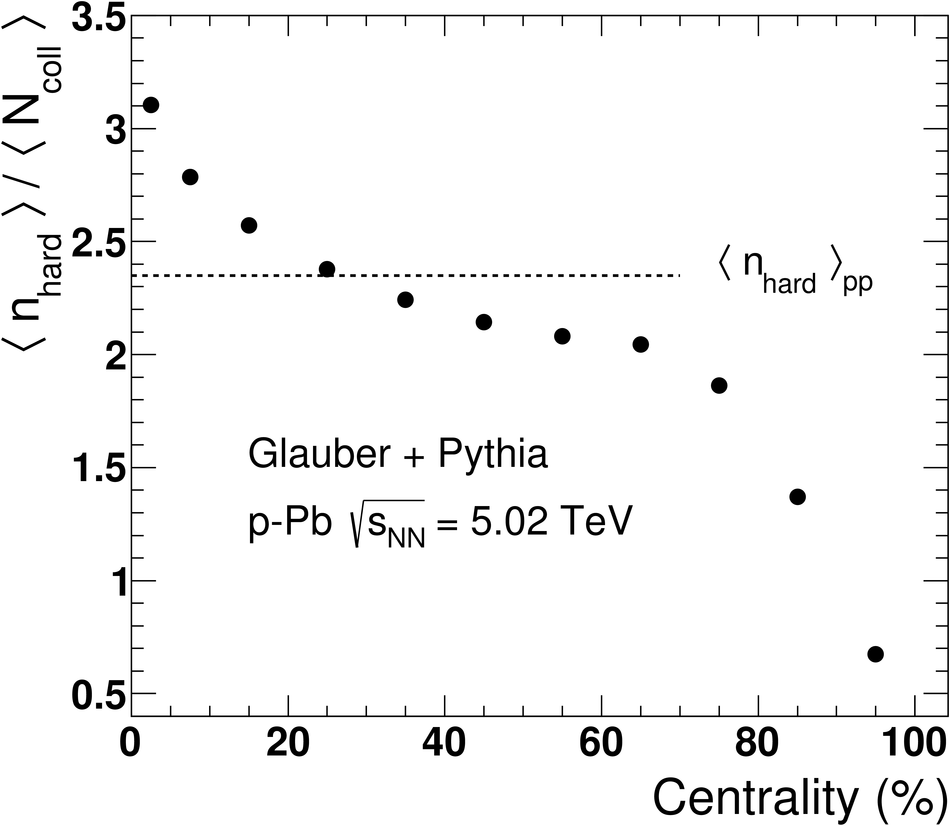## Figure 10

 Multiplicity distribution used as centrality estimators in p-Pb collisions, compared to the distribution in pp collisions at $\sqrt{s}$ = 7 TeV. The dashed lines mark the 80% and the 60% percentile of the p-Pb cross-section respectively.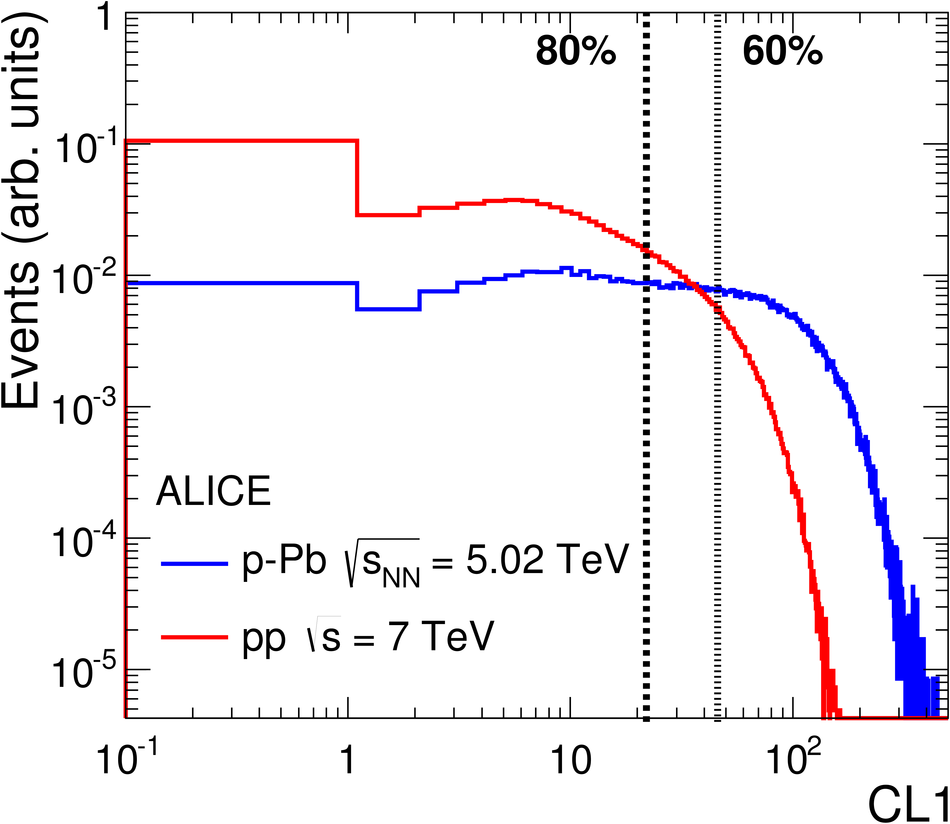## Figure 11

 Average nucleon-nucleon impact parameter as a function of the number of participants for p-Pb at $\snn$ = 5.02 TeV from a Glauber MC calculation as implemented in HIJING (no shadowing, no elastic scattering). The result depends on the modelling of the spatial parton density in the nucleon. In HIJING it is approximated by the Fourier transform of a dipole form factor.## Figure 12

 Left panel: Normalized signal from various observables (the innermost ring of VZERO-A and VZERO-C and two rapidity intervals of $\dNdeta$) versus the normalized charged-particle density averaged over $-1 < \eta < 0$. Left panel: fit with the linear function from Eq.~\ref{eq:Npartfit}. Right panel: fit with the power-law function from Eq.~\ref{eq:Plawfit}. Only data from p-Pb collisions are shown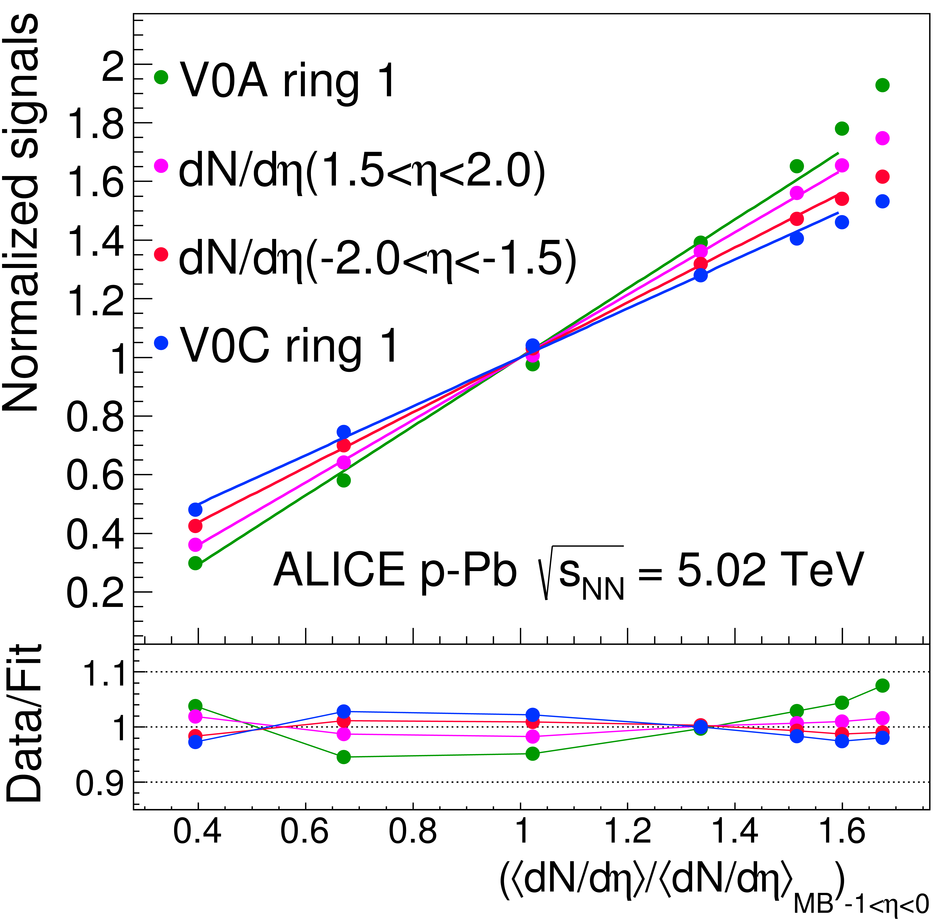## Figure 13

 Results from the fits of Eq.~\ref{eq:Npartfit} (left) and Eq.~\ref{eq:Plawfit} (right) of the normalized signals as a function of the pseudorapidity covered by the various observables. The red horizontal lines (left) indicate the ideal $N_{part}$ and $N_{coll}$ geometrical scalings. For the PHOBOS data, $\eta_{\rm cms}$ has been scaled by the ratio of the beam rapidities in p-Pb at $\sqrt{s_{\rm{NN}}}$ = 5.02 TeV and d-Au collisions at $\sqrt{s_{\rm{NN}}}$ = 200 GeV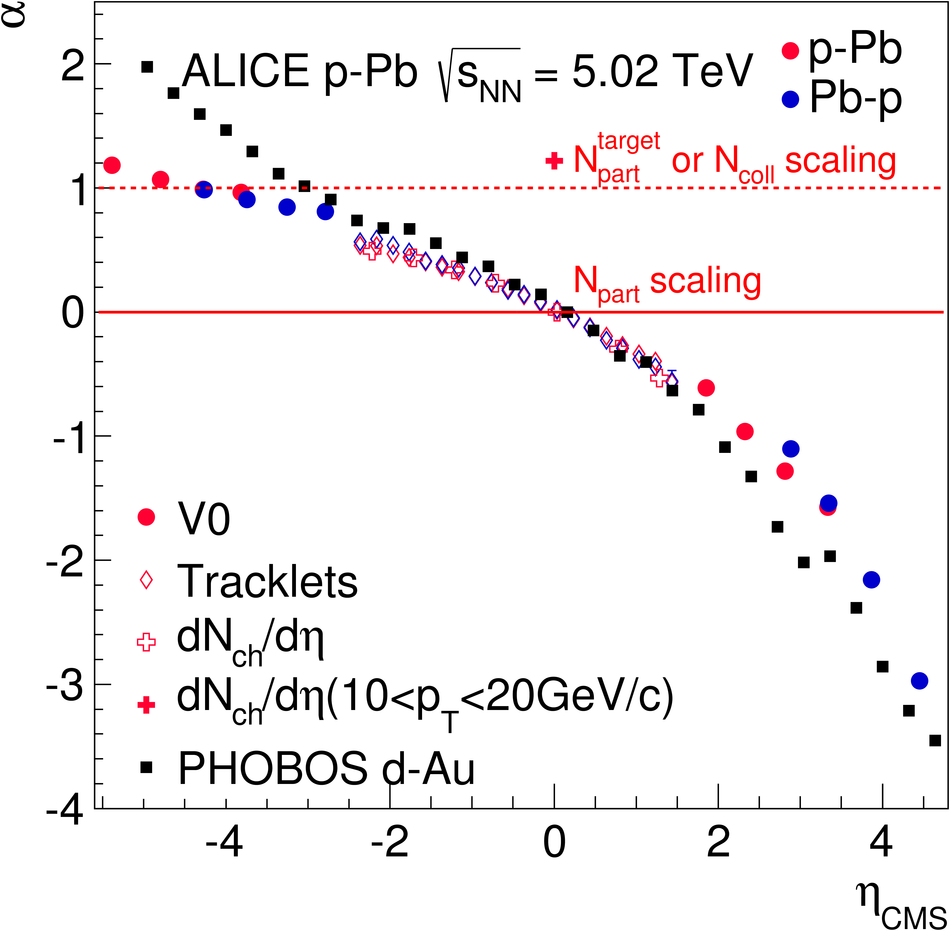## Figure 14

 $\langle \Ncoll \rangle$ values obtained with the hybrid method under the three assumptions discussed in the text. The systematic uncertainty, shown as a grey band around the $\Ncoll^{\rm mult}$ points, represents the 8% uncertainty on the $\langle \Ncoll \rangle_{\rm MB}$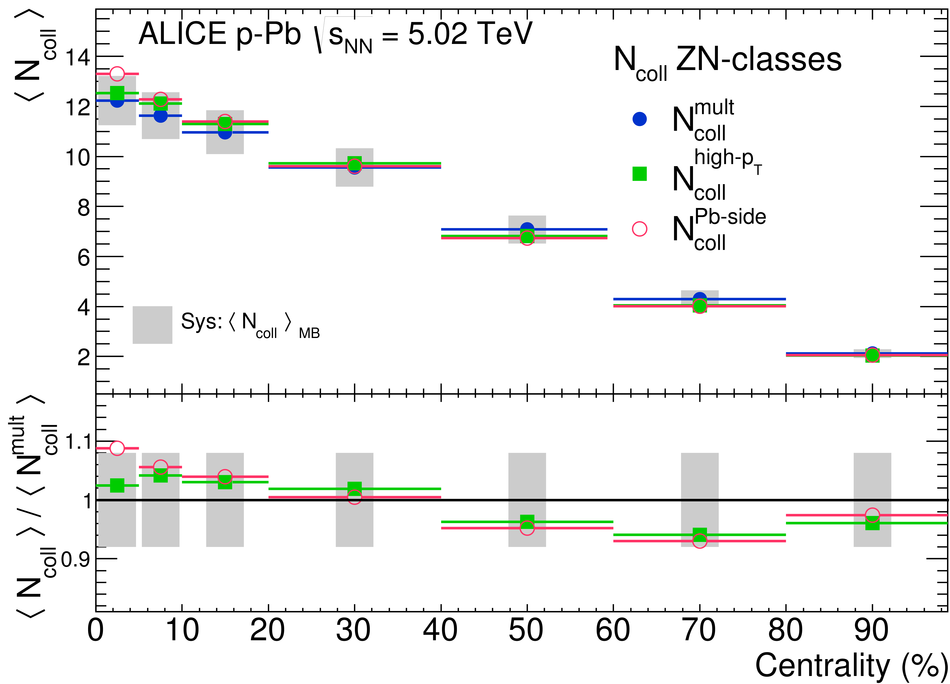## Figure 15

 Signal in the proton-going direction ZNC as a function of $\Ncoll^{\rm mult}$. The red line shows a linear fit to the first four data points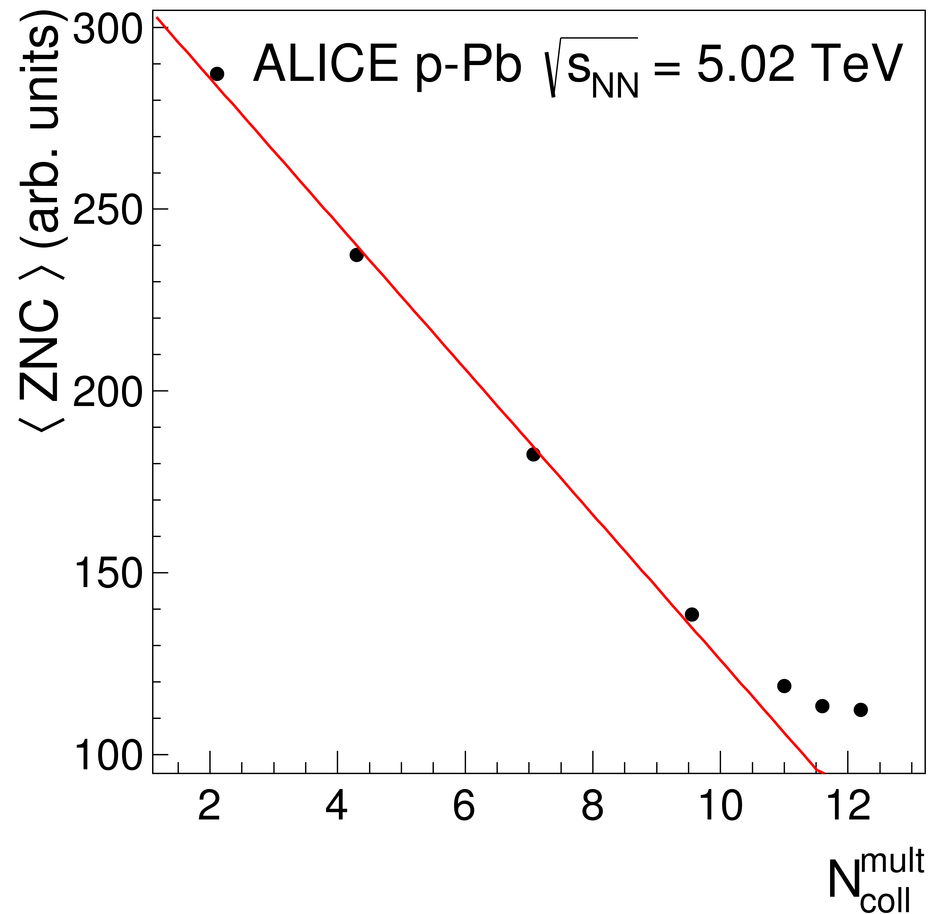## Figure 16

 Pseudorapidity density of charged particles measured in p-Pb collisions at $\snn=5.02$ TeV for various centrality classes and estimators. The different panels correspond to different centrality estimators: CL1 (top left), V0M (top right), V0A (bottom left), ZNA (bottom right)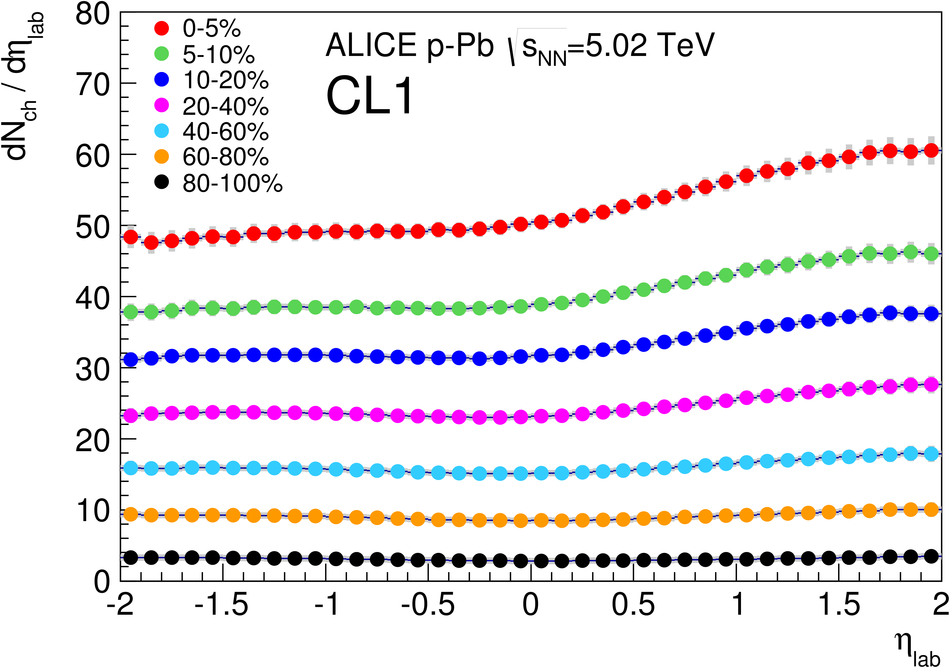## Figure 17

 Asymmetry of particle yield, calculated as ratio of the pseudorapidity density integrated in $0< \eta< 0.5$ to the one in $-1.5 < \eta < -1$ as a function of the pseudorapidity density integrated at mid-rapidity for various centrality classes and estimators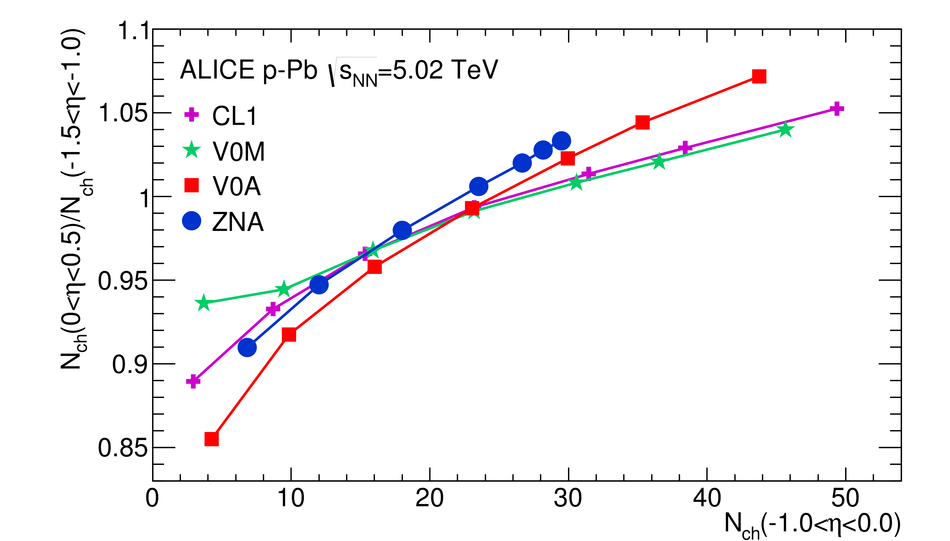## Figure 18

 Pseudorapidity density of charged particles measured in p-Pb collisions at mid-rapidity per participant as a function of $\Npart$ (left), or as a function of the mid-rapidity density (right), for various centrality estimators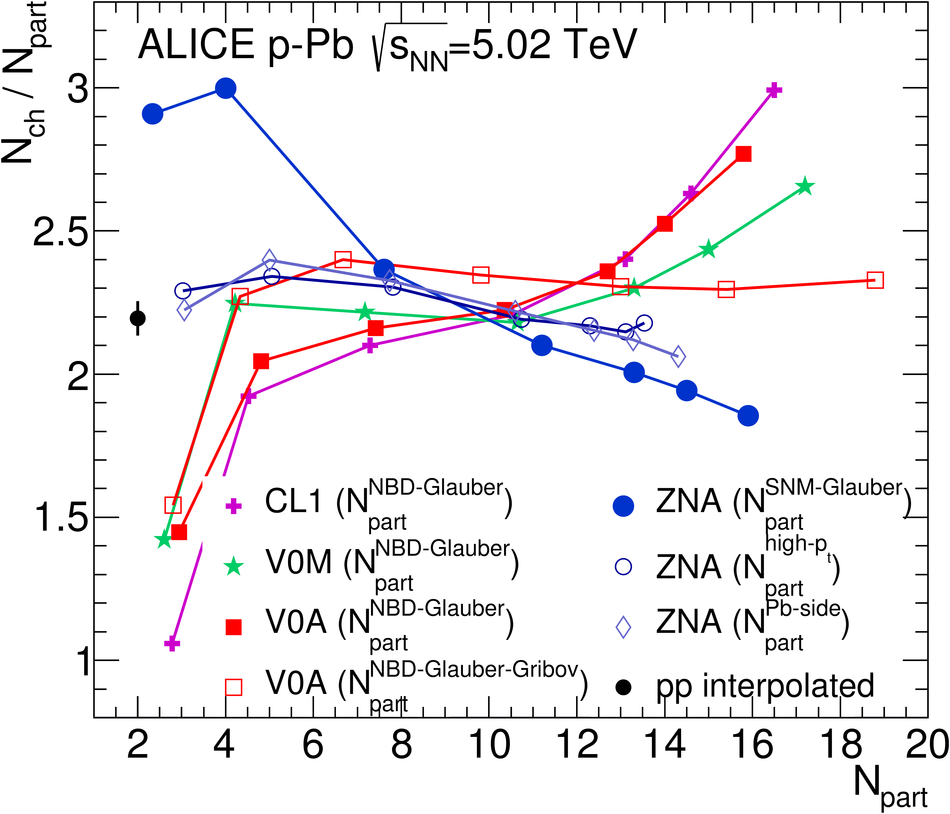## Figure 19

 $Q_{\rm pPb}$ spectra (points) of all primary charged particles for various centrality classes obtained with the different centrality estimators explained in the text. The lines are from G-PYTHIA calculations The systematic error on the spectra is only shown for the V0A 0-5% centrality bin and is the same for all others. The systematic uncertainty on pp and p-Pb normalization is shown as a gray box around unity at $\pt$ =0. The systematic uncertainty on $\langle T_{\rm pPb} \rangle_{\rm MB}$ is shown as a light blue box around unity at high $\pt$.## Figure 20

 $Q_{\rm pPb}$ spectra with the hybrid method. Spectra are calculated in ZNA classes with $\langle \Ncoll \rangle$ as given in Table \ref{tab:NcollCompareH}, and are obtained with assumptions on particle production described in Sec.~\ref{sec:hybrid}## Figure 21

 Average $Q_{\rm pPb}$ calculated ($10 < \pt < 20 \, {\rm GeV}/c$) as a function of centrality, with various centrality estimators. The left panel shows results from the data (points) and from the G-PYTHIA calculation (lines). The right panel shows the results for the hybrid method, where centrality classes are selected with ZNA, and $\langle \Ncoll \rangle$ are calculated with the assumptions on particle production described in Sec.~\ref{sec:hybrid}## Figure 22

 Left and Middle: ratio of yields in pp and p-Pb collisions for two momentum ranges, divided by the ratio of $\Nch$ in pp and p-Pb collisions. Right: ratio of the yield around 3 GeV/$c$ to the yield at high-$\pt$ Values are calculated for different centrality estimators For CL1 we also show the ratio to the value calculated with Q-PYTHIA at 3 GeV/$c$ (right panel only)